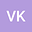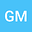Non-Fourier fractional thermoelastic two dimensional model of a hollow sphere
••Vinayak Kulkarni
University of Mumbai
Author Profile## Abstract

Assuming non-Fourier thermal effects, Tzou's dual-phase-lag model has been applied to introduce the governing heat conduction equation in the presented mathematical model. Moreover, in order to design a well-posed stable dual-phase-lag model, the governing time fractional dual-phase-lag heat equation has been established by introducing conductive temperature and thermodynamical temperature, satisfying the two-temperature theory. Due to the application of phase-lags the heat conduction equation became hyperbolic. The corresponding governing equations of motion and stresses have been considered in two-dimensional bounded spherical domain. The spherical boundaries are assumed to be traction free. The Laplace and the Legendre integral transforms have been applied to obtain the analytical solutions of conductive and thermodynamical temperatures, displacement components and thermal stresses. The Gaver-Stehfest algorithm has been employed to achieve the time domain inversions of Laplace transforms numerically, satisfying the Kuznetsov convergence criteria. Classical, fractional and generalized thermoelasticity theories has been recovered theoretically and numerically as well for various fractional orders and phase-lags values.

#### Peer review status:ACCEPTED

09 Apr 2020Submitted to Mathematical Methods in the Applied Sciences
12 Apr 2020Submission Checks Completed
12 Apr 2020Assigned to Editor
13 Apr 2020Reviewer(s) Assigned
18 Dec 2020Review(s) Completed, Editorial Evaluation Pending
18 Dec 2020Editorial Decision: Revise Minor